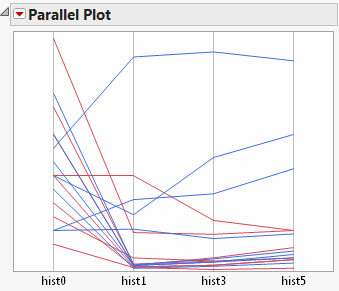Essential Graphing > Parallel Plots > The Parallel Plot
Publication date: 05/24/2021

# The Parallel Plot

To produce the plot shown in Figure 7.5, follow the instructions in Example of a Parallel Plot.

Figure 7.5 The Parallel Plot ReportA parallel plot is one of the few types of coordinate plots that show any number of variables in one plot. However, the relationships between variables might be evident only in the following circumstances:

when the variables are side by side

if you assign a color to a level of a variable to track groups

if you select lines to track groups

Tip: You can add reference lines for specification limits. For information, see Spec Limits in Using JMP.